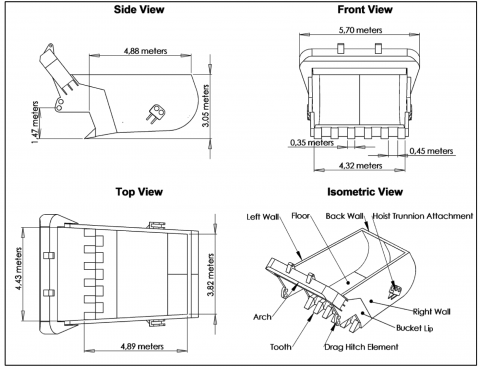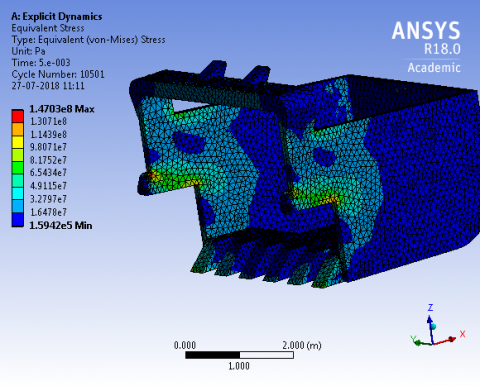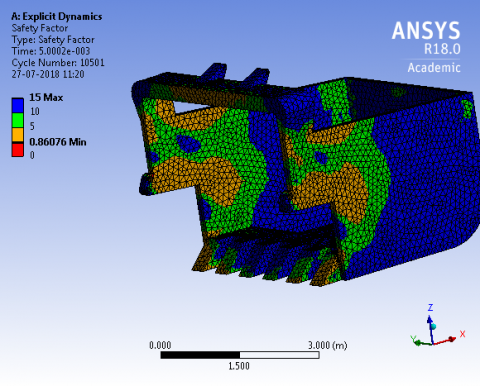# Stress Analysis of Dragline Bucket in Operating Condition

Stress Analysis of Dragline Bucket in Operating Condition

Shah Fateh Azam Piyush Rai

Indian Institute of Technology (BHU), Varanasi (UP) 221005, India

Corresponding Author Email:
shahfateh.azam29@gmail.com
Page:
10-15
|
DOI:
https://doi.org/10.18280/mmc_c.800102
4 August 2018
|
Accepted:
20 March 2019
|
Published:
31 March 2019
| Citation

OPEN ACCESS

Abstract:

Draglines are heavy and costly machines massively utilised in opencast mines to remove the overburden materials. Because of harsh operating situations advanced wear, tear, fractures and fatigue failure are generally determined in dragline. The bucket is the main component of the dragline, and it's the far supply of external loads at the equipment because of its contacts with ground material. In this study, the three-dimensional (3D) solid moving bucket models are developed, and the stress analysis has been attempted on the dragline bucket structural using finite element method (FEM). This paper investigates the stresses under maximum loading and different operating velocity conditions.

Keywords:

dragline bucket, stress analysis, factor of safety finite element method

1. Introduction

Draglines are used to do away with the overburden for exposing the minerals in a surface mine. The draglines may be greater than 4000-ton in average weight, with bucket capacities starting from 24 m3-120 m3. The buckets are dragged against the blasted muck to fill the blasted overburden. The capital cost of dragline is almost Rs 500 crore for the bucket capacity of 62 m3. A dragline bucket is a big shape that's suspended from an increase (a big truss-like structure) with cord ropes. The bucket has manoeuvred the usage of a few ropes and chains. The drag rope is used to drag the bucket assembly horizontally . By way of skillful manoeuvre of the hoist and the drag ropes, the bucket is controlled for different operations. A schematic diagram of a large dragline bucket system is shown in Figure 1. It's been determined that operational and resultant stress versions are critical troubles that cause unsteady stress to set off harm of bucket and its operational life.

In this study, dragline bucket evaluation consists of examination and expertise of the stress distribution and safety factor of the bucket frame in dynamic working conditions. Numerical modelling has been used for examining the key parameters.

Finite element analysis (FEA) has been invariably used for simulation and analysis under different loading conditions of dragline for a long time. To develop an FEA model and to simulate the cutting process of a sub-layer formation with various geometries . For the evaluation motive to evolve a 3-D finite element evaluation of soil–blade interplay primarily based on predefined horizontal and vertical failure surfaces to research the behaviour of the soil–blade interface and look at the impact of blade-cutting width and lateral boundary width on anticipated forces . To develop a numerical technique to obtain the static equilibrium state of a conventional dragline excavation system, including the static pose of the bucket, as well as internal loads are acting on an element of the excavation system . In this analysis to use the numerical method for the excavation of bucket filling and optimised in a complex granular flow [5, 6]. To study the components of the excavator to identify the problems faced while performing the hoisting and digging operation . To design the excavator bucket with force applied at the tip of teeth of the excavator bucket to find the stresses . To develop a 3D model of the bucket to determine the stresses using Abacus software .

## 1.jpgFigure 1. A dragline bucket (62m3)

2. Methodology

2.1 Numerical modeling

The finite element method (FEM) is a relatively new and effective numerical method . In this paper, the finite element method was used for simulation and analysis purpose. Finite elements are used in design improvement and optimisation purpose for any mechanical parts. In this paper, ANSYS software has been used for analysis . The simulation includes equivalent stress, deformation, and safety factor under different operating velocity conditions, prevalent in the field scale dragline operation.

2.2 The geometry of a dragline bucket

A 3D solid model of a dragline bucket was created in the AUTO CAD. The real-time dimension of a 62 m3 dragline bucket was taken for generating a solid model depicted in Figure 2.

## 2.pngFigure 2. Dragline bucket with different views 

## 3.pngFigure 3. 3D solid bucket model

The AUTO CAD generated solid model file then imported to solid works and converted to IGES file, which was then imported to ANSYS 18 to apply loading and boundary condition for simulating the dragline bucket and the existing stress environment on it .

2.3 Material property

Table1. Material properties of dragline bucket (steel) 

 Material Steel Density 7.85e-006 kg mm-3 Tensile Ultimate Strength 460 MPa Tensile Yield Strength 250 MPa Poisson's Ratio 0.3 Young's Modulus 2.e+005

In the solid model, the capacity of the bucket was 62 m3, and the mass of the bucket was 70 ton. When overburden material is filled in the bucket, then the total mass of the bucket got increased naturally. Considering sandstone as loaded material and density of sandstone varying between 1.8 to 2.4 ton/m3, the analysis was done under different operating velocity conditions .

2.4 Determination of resistive forces for different formation property

When dragline bucket is digging and moving in forwarding direction then a series of repeated formation failures occur due to the contact between the rock formation and dragline bucket. There are many analytical and empirical strategies are available for analysis of the forces that had been created for the duration of formation slicing. On this paper use the McKyes’s 2D version  Equation (1) and evaluation of the forces because of adhesion, weight, overloading, cohesion, and inertia to specific the resistance presented by way of the rock formation to earthmoving .

$\mathrm{T}=\left(\mathrm{γgd}^{2} \mathrm{N}_{\mathrm{γ}}+\mathrm{cdN}_{\mathrm{c}}+\mathrm{C}_{\mathrm{a}} \mathrm{d} \mathrm{N}_{\mathrm{ca}}+\mathrm{qdN}_{\mathrm{q}}+\mathrm{γv}^{2} \mathrm{d} \mathrm{N}_{\mathrm{a}}\right)$    (1)

where,

T = resultant cutting force

γ = formation density

d = tool depth

c = cohesion

g = gravitational acceleration

w = cutting width

v = cutting velocity of formation

Nc = cohesion coefficient

Nγ = weight coefficient

Na = inertia coefficient

reduced the equation (1) into Equation (2)

$\mathrm{T}=\left(\mathrm{γgd}^{2} \mathrm{N}_{\mathrm{γ}}+\mathrm{cdN}_{\mathrm{c}}\right)w$    (2)

By using the charts given by Hettiaratch and Reece (1974) and find out the N coefficients for the weight (γ) and cohesion (c) .

$\boldsymbol{N} \boldsymbol{x}=\boldsymbol{N}_{0}+\left(\boldsymbol{N}_{\varphi}-\boldsymbol{N}_{0}\right) \frac{\delta}{\Phi}$    (3)

Using equation (3) and (2) the estimated values of cohesion, weight coefficient and resulting cutting force are existing in Table 2. The cutting force was estimated to be 222.93 kN.

Table 2. Estimated values of input parameter

 Parameter value cohesion strength of overburden, c (kPa) 25.0 KPa Density of overburden material, γ 2.0 (t /m3) External friction angle, δ (30°) Internal friction angle, φ (40°) Cohesion coefficient, Nc 3.4 Resultant cutting force, T 222.94 KN

2.5 Finite element mesh and boundary conditions

The material in FEA modelling and simulation was steel with strengths of 460MPa, showing elastic-perfectly plastic behaviour. The meshing became performed the use of a 4-node linear tetrahedron continuum element. Figure 4 shows the resulting meshing body with an element size of 100 mm. Meshing pattern is shown in Figure 4. The resulting meshing body finds out 55148 solid elements and 14236 nodes. The boundary condition is illustrated in Figure 5.

## 4.pngFigure 4. Meshing body of bucket

## 5.pngFigure 5. Boundary condition of moving bucket

Simulation in this investigation uses the external pressure of 1.37 x10 5 Pa applied to the bucket teeth, and the velocity range is given 0.50-1.5 m/s on the bucket moving in the direction.

3. Results and Discussions

3.1 Stress distribution on the bucket

Von-mises theory has been used for the estimation of equivalent stress in the study. The von-mises yield criterion shows that yielding of a ductile material starts while the other one deviatoric stress invariant reaches an essential value . The usage of this information an engineer can say his design will fail if the maximum value of Von-mises stress prompted in the material is gr2eater than the strength of the materials. It works properly in most cases, while the material is ductile.

For this analysis, to develop a 3D solid model and simulates the formation cutting action of the dragline bucket. The modelling and analysis suggest the stress accumulation of stress in the teeth and hitch element of the moving bucket due to the failure of the bucket initiates in these areas predominantly. Find out the stress concentration regions that are most prone to failure in these conditions is critical planning for proper maintenance. The safety factor and von- Mises stress distribution variations are given below.

Case 1. When velocity was 0.5m/s, the stress value and safety factory are illustrated in Figures 6 and 7.

## 6.pngFigure 6. Von-misses stress variation on the moving bucket

## 6a.pngFigure 6a. Graph between stress and time

## 7.pngFigure 7. Safety factor variation on the moving bucket

## 7a.pngFigure 7a. Graph between safety factor and time

Case 2. When velocity was 1.0 m/s, stress value and safety factory are illustrated in Figures 8 and 9.

## 8.pngFigure 8. Von-misses stress variation on the moving bucket

## 8a.pngFigure 8a. Graph between stress and time

## 9.pngFigure 9. Safety factor variation on the moving bucket

## 9a.pngFigure 9a. Graph between safety factor and time

Case 3. When velocity was 1.50 m/ s, stress value and safety factory are illustrated in Figures 10 and 11.

## 10.pngFigure 10. Von-misses stress variation on the moving bucket

## 10a.pngFigure 10a. Graph between stress and time

## 11.pngFigure 11. Safety factor variation on the moving bucket

## 11a.pngFigure 11a. Graph between safety factor and time

Table 3. Values of stresses and safety factor with different operating velocity

 S.NO. Bucket Velocity (m/s) Stress Value (Max) (MPa) Safety Factor (Min) 1. 0.5 147.03 1.70 2. 1.0 290.44 0.84 3. 1.5 433.50 0.57

Initially when the bucket moves forward for digging the muck immediately after its ground placement, then bucket velocity is 0.5 m/s. During moving and filling in the blasted muck the bucket velocity increases to 1.0 m/s and finally, it attains a maximum filling velocity of 1.5 m/s.

From this simulation results, it is quite clear that when the magnitude of bucket velocity increases, from 0.5 to 1.5 m/s, then stress value also increases, and the maximum value of stress obtained was 433.50 MPa. This maximum stress value is close to the maximum tensile strength of steel. Furthermore, with an increase in bucket velocity, the bucket safety factor was also found to be minimum with a value of 0.57 near the teeth and hitch element of the bucket.

4. Conclusions

The simulation results show the stress value and safety factor near the hitch elements and bucket teeth. In moving conditions of the dragline bucket, it has been observed that when the velocity of bucket changes then the stress concentration value also change near the hitch elements and the teeth of the bucket, it means the chances of failure is more dominant in these locations. In the Figures shown that when increases the velocity of the bucket then increases the stresses value and decreases the safety factor value near the hitch element and teeth. This stress value isn't enough to cause the failure of the complete dragline bucket frame.

For future works, simulation of the complete dragline system under different working and loading conditions.

References

 Azam, S.F., Rai, P. (2018). Modelling of dragline bucket for determination of stress. ASME Journals, 78: 392-402.

 Mouazen, A.M., Nemenyi, M. (1999). Finite element analysis of subsoiler cutting in non-homogeneous. Soil and Tillage Research, 51(1-2): 1-15. https://doi.org/10.1016/S0167-1987(99)00015-X

 Abo-Elnor, M., Hamilton, R., Boyle, J.T. (2004). Simulation of soil–blade interaction for sandy soil using advanced 3D finite element analysis. Soil & Tillage Research, 75(1): 61-73. https://doi.org/10.1016/S0167-1987(03)00156-9

 Costello, M., Kyle, J. (2004). A method for calculating static conditions of a dragline excavation system using dynamic simulation. Mathematical and Computer Modelling, 40(3-4): 233-247. https://doi.org/10.1016/j.mcm.2004.03.001

 Coetzee, C.J., Els, D.N.J. (2009). The numerical modelling of excavator bucket filling using DEM. J. Terramechanics, 46(5): 217-227. https://doi.org/10.1016/j.jterra.2009.05.003

 Coetzee, C.J., Els, D.N.J., Dymond, G.F. (2010). Discrete element parameter calibration and the modelling of dragline bucket filling. Journal of Terramechanics, 47(1): 33-44. https://doi.org/10.1016/j.jterra.2009.03.003

 Bende, S.B., Awate, N.P. (2013). Design, modelling and analysis of excavator arm. International Journal of Design Manuf. Technology, 4: 14-20.

 Tupkar, M.P., Zaveri, S.R. (2015). Design and analysis of an excavator bucket. International Journal of Science Research Engineering & Technology, 4(3): 227-229.

 Gölbaşi, O., Demirel, N. (2015). Investigation of stress in an earthmover bucket using finite element analysis. A generic model for draglines. Journal of the Southern African Institute of Mining and Metallurgy, 115(7): 623-628. https://doi.org/10.17159/2411-9717/2015/v115n7a8

 Abo-Elnor, M., Hamilton, R., Boyle, J.T. (2003). 3D Dynamic analysis of soil-tool interaction using the finite element method. J. Terramechanics, 40(1): 51-62. https://doi.org/10.1016/j.jterra.2003.09.002

 McKyes, E. (1985). Soil Cutting and Tillage. Developments in Agricultural Engineering, Elsevier, Amsterdam, 7: 1-217.